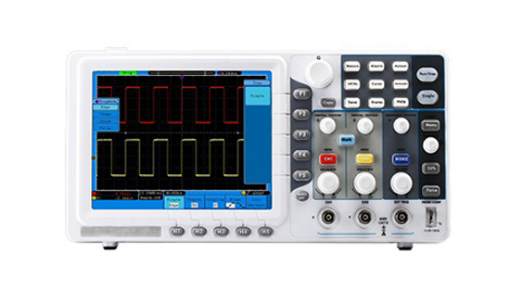# How to Choose an Oscilloscope?

An oscilloscope is a laboratory instrument commonly used to display and analyze the waveform of electronic signals. In effect, the device draws a graph of the instantaneous signal voltage as a function of time. An excellent oscilloscope for testing and analysis, an oscilloscope is a must-have for many industries and applications such as RF design, general electronic circuit design, electronics manufacturing, service, repair, and more.

But how do you know which oscilloscope is right for your application? There are 7 factors to consider when you buy an oscilloscope.### 1. Oscilloscope bandwidth

The bandwidth determines the ability of the oscilloscope to measure analog signals, which determines the maximum frequency that the instrument can accurately measure. Bandwidth is also a key determinant of price.

Determine your needs before choosing an oscilloscope. For example, a 100 MHz oscilloscope is typically guaranteed to have less than 30% attenuation at 100 MHz. To ensure better than 2% amplitude accuracy, the input should be below 20MHz.

Using the "rule of five" when choosing bandwidth. The oscilloscope bandwidth is greater than or equal to five times the maximum frequency you want, if the bandwidth is too low, your oscilloscope will not be able to resolve high-frequency changes.

A basic oscilloscope typically has a range of 50 MHz to 200 MHz. If you need more bandwidth, you can use a higher-performance oscilloscope to cover beyond 350 MHz and reach tens of GHz.

### 2. Oscilloscope sampling rate

Sample rate (samples per second) is the rate at which the oscilloscope samples the signal, similar to the frame rate of a camera; this determines how much waveform detail the oscilloscope can capture.

Again, we recommend using the "rule of five": use at least 5 times the sampling rate of your circuit's highest frequency components. Most basic oscilloscopes have a (maximum) sample rate of 1 to 2 GS/s. Remember that basic oscilloscopes have bandwidths up to 200MHz, so scope designers typically design scopes with 5 to 10 times oversampling at maximum bandwidth.

The faster the sampling rate, the less information will be lost and the more effectively the oscilloscope will be able to represent the signal under test; however, it will also fill up the memory faster, which in turn limits the amount of time that data can be acquired.

Most entry-level oscilloscopes have a maximum sample rate of 1 to 2 GS/s, while mid-range oscilloscopes can have a maximum sample rate of 5 to 10 GS/s.

### 3. Oscilloscope channel

Oscilloscopes use analog channels to store and display signals. In general, the more channels the better, although adding channels will increase the price.

Whether you want to choose 2 or 4 analog channels will depend on your application. For example, you can use two channels to compare the input and output of components. Four analog channels allow you to compare more signals and provide greater flexibility to combine channels mathematically (for example, multiply for power, or subtract for differential signals).

### 4. Oscilloscope probes

Good metrology starts with the probe tip. Oscilloscopes and probes work together as a system, so be sure to consider probes when choosing an oscilloscope.

During the measurement, the probe actually becomes part of the circuit, introducing resistive, capacitive and inductive loads (changing measurements). To minimize the effect, it is best to use the special probes that come with the oscilloscope. A wide range of compatible probes will allow you to use your oscilloscope in more applications.

In addition, it is also important to choose a passive probe with sufficient bandwidth. The bandwidth of the probe should match the bandwidth of the oscilloscope.

### 5. Oscilloscope trigger

Triggering provides a stable display, allowing you to adjust zeroing on specific portions of complex waveforms. All oscilloscopes offer edge triggering, and most offer pulse width triggering. And the wider the range of trigger options available to an oscilloscope, the more flexible the oscilloscope (and the faster you'll find the source of your problems!)

### 6. Oscilloscope record length

The record length is the number of points in a complete waveform record. In general, oscilloscopes can only store a finite number of samples, so the longer the record length, the better.

Acquisition time = record length/sampling rate

So, with a record length of 1M points and a sampling rate of 250 MS/s, the oscilloscope will capture 4 ms. A good basic oscilloscope typically stores over 2,000 points, which is more than enough for a stable sine wave signal (maybe 500 points). However, to find out the cause of timing anomalies in complex digital data streams, the record length of more than 1M points should be considered.

Oscilloscopes with record lengths in the millions of points can display many pictures of the signal activity, an essential feature for studying complex waveforms.

### 7. Oscilloscope Automatic measurement and analysis

Automated waveform measurements make it easier to get accurate numerical readings.

Most oscilloscopes provide front-panel buttons and/or on-screen menus for accurate automated measurements, including amplitude, period, and rise/fall time. Many digital oscilloscopes also offer average and RMS calculations, duty cycles and other mathematical operations.

For example, the channel calculation function allows you to perform operations such as addition, subtraction, and multiplication on waveforms. Use the waveform multiplication function to multiply voltage and current to obtain power values; use the subtraction function to roughly estimate differential measurements. The Fast Fourier Transform (FFT) function will allow you to view the frequency spectrum of the captured waveform.